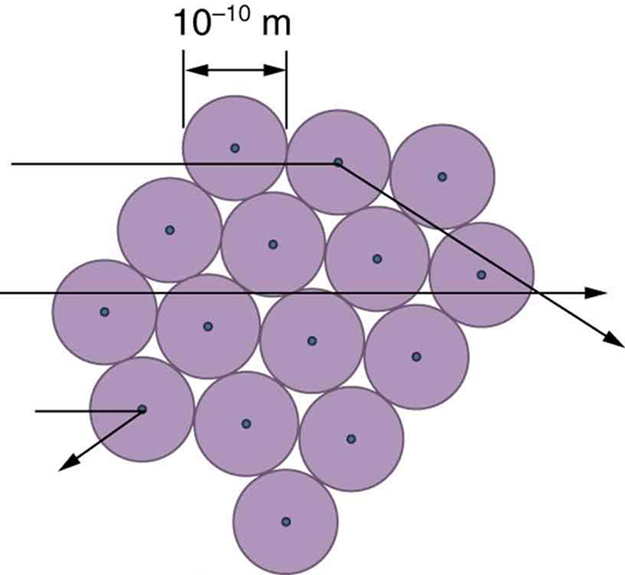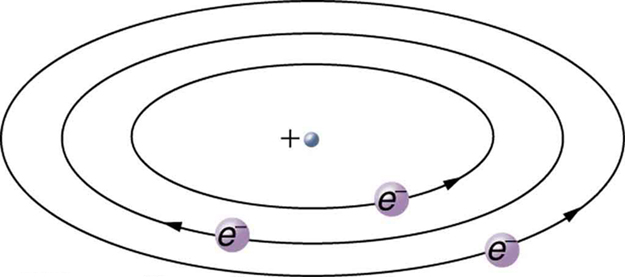# 30.2 Discovery of the parts of the atom: electrons and nuclei  (Page 5/7)

 Page 5 / 7

Although the results of the experiment were published by his colleagues in 1909, it took Rutherford two years to convince himself of their meaning. Like Thomson before him, Rutherford was reluctant to accept such radical results. Nature on a small scale is so unlike our classical world that even those at the forefront of discovery are sometimes surprised. Rutherford later wrote: “It was almost as incredible as if you fired a 15-inch shell at a piece of tissue paper and it came back and hit you. On consideration, I realized that this scattering backwards ... [meant] ... the greatest part of the mass of the atom was concentrated in a tiny nucleus.” In 1911, Rutherford published his analysis together with a proposed model of the atom. The size of the nucleus was determined to be about ${\text{10}}^{-\text{15}}\phantom{\rule{0.25em}{0ex}}\text{m}$ , or 100,000 times smaller than the atom. This implies a huge density, on the order of ${\text{10}}^{\text{15}}\phantom{\rule{0.25em}{0ex}}{\text{g/cm}}^{3}$ , vastly unlike any macroscopic matter. Also implied is the existence of previously unknown nuclear forces to counteract the huge repulsive Coulomb forces among the positive charges in the nucleus. Huge forces would also be consistent with the large energies emitted in nuclear radiation.

The small size of the nucleus also implies that the atom is mostly empty inside. In fact, in Rutherford’s experiment, most alphas went straight through the gold foil with very little scattering, since electrons have such small masses and since the atom was mostly empty with nothing for the alpha to hit. There were already hints of this at the time Rutherford performed his experiments, since energetic electrons had been observed to penetrate thin foils more easily than expected. [link] shows a schematic of the atoms in a thin foil with circles representing the size of the atoms (about ${\text{10}}^{-\text{10}}\phantom{\rule{0.25em}{0ex}}\text{m}$ ) and dots representing the nuclei. (The dots are not to scale—if they were, you would need a microscope to see them.) Most alpha particles miss the small nuclei and are only slightly scattered by electrons. Occasionally, (about once in 8000 times in Rutherford’s experiment), an alpha hits a nucleus head-on and is scattered straight backward.An expanded view of the atoms in the gold foil in Rutherford’s experiment. Circles represent the atoms (about 10 − 10 m size 12{"10" rSup { size 8{ - "10"} } " m"} {} in diameter), while the dots represent the nuclei (about 10 − 15 m size 12{"10" rSup { size 8{ - "15"} } " m"} {} in diameter). To be visible, the dots are much larger than scale. Most alpha particles crash through but are relatively unaffected because of their high energy and the electron’s small mass. Some, however, head straight toward a nucleus and are scattered straight back. A detailed analysis gives the size and mass of the nucleus.

Based on the size and mass of the nucleus revealed by his experiment, as well as the mass of electrons, Rutherford proposed the planetary model of the atom    . The planetary model of the atom pictures low-mass electrons orbiting a large-mass nucleus. The sizes of the electron orbits are large compared with the size of the nucleus, with mostly vacuum inside the atom. This picture is analogous to how low-mass planets in our solar system orbit the large-mass Sun at distances large compared with the size of the sun. In the atom, the attractive Coulomb force is analogous to gravitation in the planetary system. (See [link] .) Note that a model or mental picture is needed to explain experimental results, since the atom is too small to be directly observed with visible light.Rutherford’s planetary model of the atom incorporates the characteristics of the nucleus, electrons, and the size of the atom. This model was the first to recognize the structure of atoms, in which low-mass electrons orbit a very small, massive nucleus in orbits much larger than the nucleus. The atom is mostly empty and is analogous to our planetary system.

Rutherford’s planetary model of the atom was crucial to understanding the characteristics of atoms, and their interactions and energies, as we shall see in the next few sections. Also, it was an indication of how different nature is from the familiar classical world on the small, quantum mechanical scale. The discovery of a substructure to all matter in the form of atoms and molecules was now being taken a step further to reveal a substructure of atoms that was simpler than the 92 elements then known. We have continued to search for deeper substructures, such as those inside the nucleus, with some success. In later chapters, we will follow this quest in the discussion of quarks and other elementary particles, and we will look at the direction the search seems now to be heading.

## Phet explorations: rutherford scattering

How did Rutherford figure out the structure of the atom without being able to see it? Simulate the famous experiment in which he disproved the Plum Pudding model of the atom by observing alpha particles bouncing off atoms and determining that they must have a small core.

## Section summary

• Atoms are composed of negatively charged electrons, first proved to exist in cathode-ray-tube experiments, and a positively charged nucleus.
• All electrons are identical and have a charge-to-mass ratio of
$\frac{{q}_{e}}{{m}_{e}}=-\text{1.76}×{\text{10}}^{\text{11}}\phantom{\rule{0.25em}{0ex}}\text{C/kg.}$
• The positive charge in the nuclei is carried by particles called protons, which have a charge-to-mass ratio of
$\frac{{q}_{p}}{{m}_{p}}=9\text{.}\text{57}×{\text{10}}^{7}\phantom{\rule{0.25em}{0ex}}\text{C/kg}\text{.}$
• Mass of electron,
${m}_{e}=9\text{.}\text{11}×{\text{10}}^{-\text{31}}\phantom{\rule{0.25em}{0ex}}\text{kg}\text{.}$
• Mass of proton,
${m}_{p}=1\text{.}\text{67}×{\text{10}}^{-\text{27}}\phantom{\rule{0.25em}{0ex}}\text{kg.}$
• The planetary model of the atom pictures electrons orbiting the nucleus in the same way that planets orbit the sun.

## Conceptual questions

What two pieces of evidence allowed the first calculation of ${m}_{e}$ , the mass of the electron?

(a) The ratios ${q}_{e}/{m}_{e}$ and ${q}_{p}/{m}_{p}$ .

(b) The values of ${q}_{e}$ and ${E}_{B}$ .

(c) The ratio ${q}_{e}/{m}_{e}$ and ${q}_{e}$ .

How do the allowed orbits for electrons in atoms differ from the allowed orbits for planets around the sun? Explain how the correspondence principle applies here.

## Problem exercises

Rutherford found the size of the nucleus to be about ${\text{10}}^{-\text{15}}\phantom{\rule{0.25em}{0ex}}\text{m}$ . This implied a huge density. What would this density be for gold?

$\text{6}×{\text{10}}^{\text{20}}\phantom{\rule{0.25em}{0ex}}{\text{kg/m}}^{3}$

In Millikan’s oil-drop experiment, one looks at a small oil drop held motionless between two plates. Take the voltage between the plates to be 2033 V, and the plate separation to be 2.00 cm. The oil drop (of density $0\text{.}{\text{81 g/cm}}^{3}$ ) has a diameter of $4\text{.}0×{\text{10}}^{-6}\phantom{\rule{0.25em}{0ex}}\text{m}$ . Find the charge on the drop, in terms of electron units.

(a) An aspiring physicist wants to build a scale model of a hydrogen atom for her science fair project. If the atom is 1.00 m in diameter, how big should she try to make the nucleus?

(b) How easy will this be to do?

(a) $\text{10.0 μm}$

(b) It isn’t hard to make one of approximately this size. It would be harder to make it exactly $\text{10.0 μm}$ .

Explain why a building made of bricks has smaller entropy than the same bricks in a disorganized pile. Do this by considering the number of ways that each could be formed (the number of microstates in each macrostate).
Omo physics hard ooo 😂😭😭😭
yeah but just need patient
Musbahu
The closeness of a measurement to the accepted value for a specific physical quantity is known as what ?
what's the procedure for solving exercise 9.
If you dive into water, you reach greater depths than if you do a belly flop. Explain this difference in depth using the concept of conservation of energy. Explain this difference in depth
A mass of 10kg move with a volocity of 4mß.find it's kinetic energy
80m/s²
Angel
KE=1/2mv²
Angel
m= 10
Angel
v=4
Angel
Happy to help
Angel
hi
Dike
What is the unit of force in F.P.S system
f=ma, f=m/s2
MD
lb-ft/s^2
Mary
what is an F.P.S system
Angel
please someone should send his or her number o. this platform
Pelumi
what is the unit for force
newtons
Angel
hello
Pelumi
Newton or kg. m/sec^2
Hiba
v=sin(att/s) Prove please as dimension consistent or not
v=sin(att/s) Prove please as dimension consistent or not
Hashim
what is physics
what is physic and what are its branches
= Kinematics = Quantum Physics = Dynamics = Statistics = Linear algebra = Calculus (Pre - Calculus, Calculus I Calculus II Calculus III Calculus IV) =Astrophysics And many more...... .....
puvananathan
what is andromeda
what is the milky way
What's the difference between deceleration and negative acceleration?
MNEMONICS FOR THE BRANCHES OF PHYSICS
are you asking for the mnemonics or you want to share it with us.?!?
paul
umar
While operating, a TV monitor is placed on its side during maintenance. The image on the monitor changes color and blurs slightly. Discuss the possible relation of these effects to the Earth’s magnetic field.
WhileGot questions? Get instant answers now! operating, a TV monitor is placed on its side during maintenance. The image on the monitor changes color and blurs slightly. Discuss the possible relation of these effects to the Earth’s magnetic field.
Dedachew

#### Get Jobilize Job Search Mobile App in your pocket Now!ByByBy OpenStaxBy Dionne MahaffeyBy OpenStaxBy OpenStaxBy Brooke DelaneyBy Alec MoffitBy Richley CrapoBy Dionne MahaffeyBy Brooke DelaneyBy Madison Christian